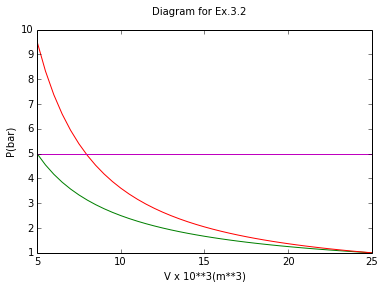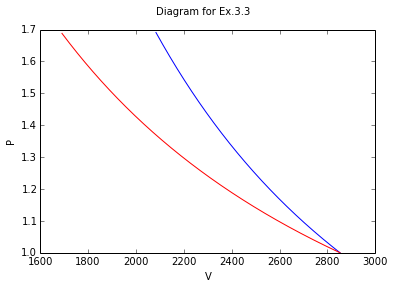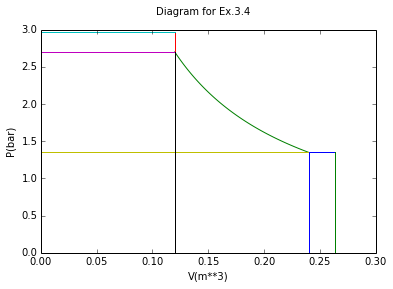# Chapter 3 : Volumetric Properties Of Pure Fluids¶

## Example 3.1 page no : 46¶

In :
import math

# Variables
P1 = 1.;			#Pressure = 1Bar
T1 = 20.;			#Temp = 293.15K(20C)
Beta = 1.487*10**(-3);			#vol expansivity(K**-1)
k = 62.*10**(-6);			#isothermal compressibility(bar**-1)
V1 = 1.287*10**(-3);			#Volume(m**3 kg**-1)

# Calculations and Results
#(a)
#Find (dP/dT)v??
#Using eq.(3.4),V consmath.tant hence dV = 0
ans_a = (Beta/k);
print '(a)The value of (dp/dT)v is ',ans_a,'K**-1'

#(b)
#Find Pressure when acetone heated at const. Vol from T1(1bar) to T2.
T2_b = 30.;			#Temp2 = 303.15K(30C)
del_P = ans_a*(T2_b-T1);
ans_b = P1+del_P;
print '(b)The pressure is ',ans_b,'bar'

#(c)
#Find vol. change when acetone changed from T1(P1) to T2(P2)
T2_c = 0;			#Temp2 = 273.15K(0C)
P2 = 10.;			#pressure = 10bar
#solve using Eq. (3.5)
ln_value = (Beta*(T2_c-T1))-(k*(P2-P1));			#ln(V2/V1)
ratio = math.exp(ln_value);			#taking antimath.log,V2/V1
V2 = ratio*V1;
del_V = round(V2-V1,6)
print '(c)The change in Volume is ',del_V*1000,'(X 10**-3) m**3 kg**-1'

(a)The value of (dp/dT)v is  23.9838709677 K**-1
(b)The pressure is  240.838709677 bar
(c)The change in Volume is  -0.038 (X 10**-3) m**3 kg**-1


## Example 3.2 page no : 47¶

In :
%matplotlib inline

import math
from numpy import linspace
from matplotlib.pyplot import plot,suptitle,xlabel,ylabel

# Variables
#Figure
P = [1, 5];
V = [25 ,25];

# Calculations and Results
plot(V,P)
V = linspace(5,25,41)
P = 25*V**-1;
plot(V,P)
P = P**1.4;
plot(V,P)
P = [5 ,9.52];
V = [5 ,5];
plot(V,P) #,rect = [0,0,30,10])
suptitle("Diagram for Ex.3.2")
xlabel("V x 10**3(m**3)")
ylabel("P(bar)")
P = [5, 5];
V = [5 ,25];
plot(V,P)

#Initial Stage
P1 = 1.;			#Pressure = 1bar
T1 = 298.15;			#Temp1 = 298.15K(25C)

#Final Stage
P2 = 5.;			#Pressure = 1bar
#Temp same as Temp1(Isothermal)

R = 8.314;			#J/Mol/K
Cv = (5./2)*R;			#J/Mol/K
Cp = (7./2)*R;			#J/Mol/K

#(a)
#Const Vol follwd by const Pressure
T2 = T1*(P2/P1);
#By Eq 2.23
del_T = T2-T1;
Q1 = Cv*(T2-T1);			#Heat at const Vol
Q2 = Cp*(T1-T2);			#Heat at const pressure

Q_a = round(Q1+Q2);
W_a = -Q_a;			#W = del_U-Q,here del_U = 0
print ('       (a) Heating at consmath.tant volume Followed by cooling at consmath.tant Pressure')
print 'work done by heating at const vol followed by const Pressure ',W_a,'J'
print 'Heat Transferred Q ',Q_a,'J'
print ('change in Internal Energy and enthalpy  =  0')

#(b)
#Isothermal Compression
#By Eq. (3.26)
Q_b = round(R*T1*math.log(P1/P2));
W_b = -Q_b;
print ('       (b) Isothermal compression')
print 'work done by Isothermal compression ',W_b,'J'
print 'Heat Transferred Q',Q_b,'J'
print ('change in Internal Energy and enthalpy  =  0')

#(c)
gama = Cp/Cv;
V1 = (R*T1)/(P1**(10**5));
V2 = V1*(P1/P2);
T2_c = T1*((V1/V2)**(gama-1));			#Kelvin(K)
P2_c = P1*((V1/V2)**gama);			#bar
#Umath.sing Eq. (3.31)
W_c = round(Cv*(T2_c-T1));			#W = Cv*del_T(Joules)
Q_c = -W_c;

print ('       (c) Adiabatic compression followed by cooling at consmath.tant Volume')
print 'work done by Adiabatic compression Followed by Cooling at const Vol ',W_c,'J'
print 'Heat Transferred Q',Q_c,'J'
print 'change in Internal Energy and enthalpy  =  0'

       (a) Heating at consmath.tant volume Followed by cooling at consmath.tant Pressure
work done by heating at const vol followed by const Pressure  9915.0 J
Heat Transferred Q  -9915.0 J
change in Internal Energy and enthalpy  =  0
(b) Isothermal compression
work done by Isothermal compression  3990.0 J
Heat Transferred Q -3990.0 J
change in Internal Energy and enthalpy  =  0
(c) Adiabatic compression followed by cooling at consmath.tant Volume
work done by Adiabatic compression Followed by Cooling at const Vol  5600.0 J
Heat Transferred Q -5600.0 J
change in Internal Energy and enthalpy  =  0## Example 3.3 page no : 48¶

In :
%matplotlib inline

from matplotlib.pyplot import plot,suptitle,xlabel,ylabel
import math
from numpy import linspace

# Variables
#Figure
V = linspace(2083,2853,1541);
P = 2853*V**-1;
P = P**1.67;
plot(V,P)
P = [1.698, 1.698];
V = [1690 ,2083];
plot(V,P)
V = linspace(1690,2853,2327)
P = 2853*V**-1;
plot(V,P)
suptitle("Diagram for Ex.3.3")
xlabel("V")
ylabel("P")

# Variables for the Ideal Gas
R = 8.314;			#J/Mol/K
Cv = (3./2)*R;			#J/Mol/K
Cp = (5./2)*R;			#J/Mol/K
gama = Cp/Cv;

# Calculations and Results

#(a)
P1 = 1;			#Pressure = 1bar
T1 = 343.15;			#Temp1 = 343.15K(70C)
T2 = 423.15;			#Temp2 = 423.15K(150C)
del_U_a = (Cv*(T2-T1));
W_a = del_U_a;
del_H_a = (Cp*(T2-T1));
#Umath.sing Eq. (3.29b)
P2 = P1*((T2/T1)**(gama/(gama-1)));			#bar

#(b)
#cooled form 150C to 70C at Const pressure
#Umath.sing Eq.(3.27)
Q_b = round(Cp*(T1-T2));
del_H_b = Q_b;
#for Ideal Gas
del_U_b = round(Cv*(T1-T2));
#by First law
W_b = del_U_b-Q_b;			#Joules

#(c)
#Expanded Isothermally to original state
del_U_c = 0;			#isothermal
del_H_c = 0;			#isothermal
Q_c = round(R*T1*math.log(P2/P1));
W_c = -Q_c;

#Entire process
Qt  =  Q_a+Q_b+Q_c;
Wt  =  W_a+W_b+W_c;
del_Ut = del_U_a+del_U_b+del_U_c;
del_Ht = del_H_a+del_H_b+del_H_c;

#PartII(Irreversible)
eta = .80;			#Efficiency = 80%

#(a)
Wm_a = (W_a/eta);
Qm_a = del_U_a-Wm_a;			#del_U remains same (by First Law)

#(b)
Wm_b = round(W_b/eta);
Qm_b = del_U_b-Wm_b;			#del_U remains same (by First Law)

#(c)
Wm_c = round(W_c*eta);
Qm_c = del_U_c-Wm_c;			#del_U remains same (by First Law)

#Entire Process
Qmt  =  Qm_a+Qm_b+Qm_c;
Wmt  =  Wm_a+Wm_b+Wm_c;

del_U_rev = [del_U_a,del_U_b,del_U_c];
del_H_rev = [del_H_a,del_H_b,del_H_c];
Qrev = [Q_a,Q_b,Q_c];
Wrev = [W_a,W_b,W_c];
Sumr = [del_Ut,del_Ht,Qt,Wt];

del_U_irev = del_U_rev;
del_H_irev = del_H_rev;
Qirev = [Qm_a,Qm_b,Qm_c];
Wirev = [Wm_a,Wm_b,Wm_c];
Sumi = [del_Ut,del_Ht,Qmt,Wmt];

print ('     (b)Cooled form 150C to 70C at Const pressure')
print ('     (c)Expanded Isothermally to original state')

print ('   Mechanically reversible');

Ans_rev = [del_U_rev,del_H_rev,Qrev,Wrev];

print '   del U   del H     Q        W',Ans_rev,'Sum',Sumr
print ('        Irreversible');

Ans_irev = [del_U_irev,del_H_irev,Qirev,Wirev];

print '   del U   del H     Q        W',Ans_irev,'Sum',Sumi

     (a)Adiabatic Compression
(b)Cooled form 150C to 70C at Const pressure
(c)Expanded Isothermally to original state
Mechanically reversible
del U   del H     Q        W [[997.6800000000001, -998.0, 0], [1662.8, -1663.0, 0], [0, -1663.0, 1495.0], [997.6800000000001, 665.0, -1495.0]] Sum [-0.31999999999993634, -0.20000000000004547, -168.0, 167.68000000000006]
Irreversible
del U   del H     Q        W [[997.6800000000001, -998.0, 0], [1662.8, -1663.0, 0], [-249.41999999999985, -1829.0, 1196.0], [1247.1, 831.0, -1196.0]] Sum [-0.31999999999993634, -0.20000000000004547, -882.4200000000001, 882.0999999999999]## Example 3.4 page no : 49¶

In :
%matplotlib inline

from matplotlib.pyplot import plot,suptitle,xlabel,ylabel
from numpy import linspace
import math

#Figure
P = [1.35 ,1.35];
V = [0.24, 0.264];
plot(V,P)
V = linspace(0.12,0.24,121)
P = 0.324*V**-1;
plot(V,P)
P = [2.7, 2.97];
V = [0.12, 0.12];
plot(V,P)
suptitle("Diagram for Ex.3.4")
xlabel("V(m**3)")
ylabel("P(bar)")

P = [2.97, 2.97];
V = [0 ,0.12];
plot(V,P)
P = [2.7, 2.7];
V = [0 ,0.12];
plot(V,P)
P = [1.35, 1.35];
V = [0 ,0.24];
plot(V,P)
P = [0, 2.7];
V = [0.12, 0.12];
plot(V,P)
P = [0 ,1.35];
V = [0.24, 0.24];
plot(V,P)
P = [0 ,1.35];
V = [0.264, 0.264];
plot(V,P)

# Variables
m = 0.4;			#Kg
M = 28.;			#Molecular Mass Of Nitrogen
T1 = 300.15;			#Temp = 300.15K(27C)
Pn = 0.35;			#Pressure of nitrogen  =  0.35bar
Pa = 1.;			#Atm Pressure  =  1bar
R = 8.314;			#J/Mol/K
Cv = (5./2)*R;			#J/Mol/K
Cp = (7./2)*R;			#J/Mol/K
gama = Cp/Cv;

n = (m/M)*1000;			#moles

# Calculations and Results

#(a)
#Immersed In ice/water bath
T2 = 273.15;			#Temp = 273.15K(0C)
W_a = -round(n*R*(T2-T1));			#Joules
del_H_a = round(Cp*(T2-T1),0);
Q_a =  (n*del_H_a);
del_U_a = round((Q_a+W_a)/n,0);
print ('(a)Immersed In ice/water bath')
print 'work done ',W_a,'J'
print 'Heat Transferred Q  =  ',Q_a,'J'
print 'change in Internal Energy ',del_U_a,'J'
print 'change in enthalpy ',del_H_a,'J'

#(b)
#Isothermal Compression
del_U_b = 0;			#Isothermal
del_H_b = 0;			#Isothermal
W_b = -round(n*R*T2*math.log(1./2));			#W = nRTln(V3/V2),here V3/V2 = 0.5(Given)
Q_b = -W_b;
print ('(b)Isothermal Compression')
print 'work done by Isothermal Compression ',W_b,'J'
print 'Heat Transferred Q  =  ',Q_b,'J'
print 'change in Internal Energy ',del_U_b,'J'
print 'change in enthalpy ',del_H_b,'J'

#(c)
#constant Vol Process
W_c = 0;			#const Vol
del_H_c = round((Cp*(T1-T2))/n,0);
del_U_c = round(Cv*(T1-T2),0);
Q_c = round(n*del_U_c);
print ('(c)Consmath.tant Vol Process')
print 'work done by Const Vol Process ',W_c,'J'
print 'Heat Transferred Q  =  ',Q_c,'J'
print 'change in Internal Energy ',del_U_c,'J'
print 'change in enthalpy ',del_H_c,'J'

(a)Immersed In ice/water bath
work done  3207.0 J
Heat Transferred Q  =   -11228.5714286 J
change in Internal Energy  -562.0 J
change in enthalpy  -786.0 J
(b)Isothermal Compression
work done by Isothermal Compression  22487.0 J
Heat Transferred Q  =   -22487.0 J
change in Internal Energy  0 J
change in enthalpy  0 J
(c)Consmath.tant Vol Process
work done by Const Vol Process  0 J
Heat Transferred Q  =   8014.0 J
change in Internal Energy  561.0 J
change in enthalpy  55.0 J## Example 3.6 page no : 49¶

In :
import math

# Variables
n = 1.;			#Molar Rate(mol/s)
D = 5.;			#inner Diameter(cm)
R = 83.14;
Cp = (7./2)*R;
M = 29*10.**-3;			#Molar mass(g/mol)
T = 293.15;			#temperature = 293.15K(20C)
P1 = 6.;			#Upstream Pressure
P2 = 3.;			#Downstream Pressure

# Calculations
#from Eq.(2.24b)
A = (math.pi/4)*((D*10**-2)**2);			#Area(m**2)
#upstream molar Volume
V1 = (R*T/P1)*10**-6;			#m**3/mol
u1 = n*V1/A;			#velocity(m/s)
V2 = 2*V1;
u2 = 2*u1;
del_KE = round(n*M*((u2**2)-(u1**2))/2,3);			#J/s(W)
del_T = round(-del_KE/(Cp*0.1),4);			#K

# Results
print 'Change in KE ',del_KE,'W or J/s'
print 'Change in Temperature ',del_T,'K'

Change in KE  0.186 W or J/s
Change in Temperature  -0.0064 K


## Example 3.7 page no : 50¶

In :
import math

# Variables
T = 473.15;			#Temp = 473.15k(200C)
P = 10.;			#Pressure = 10bar
B = -0.388;			#Viral Coefficient(m**3/Kmol)
C = -26.*(10**(-3));			#Viral Coefficient(m**6/(kmol)**2)

# Calculate V and Z for isopropyl vapor
R = 83.14*(10**(-3));			#m**3bar/Kmol/K

# Calculations and Results
#(a)
#Ideal Gas equation
V_a = round((R*T)/P,3);
Z_a = 1.;			#Ideal Gas
print ('(a) By Ideal gas Equation')
print 'V  =  ',V_a,'m**3/kmol'
print 'Z  =  ',Z_a

#(b)
#Using Eqution 3.37 -> Z = PV/RT = 1+BP/RT
V_b = round((R*T/P)+B,3);
Z_b = round(P*V_b/(R*T),4);
print ('(b) Using Eqution 3.37 -> Z = PV/RT = 1+BP/RT')
print 'V  =  ',V_b,'m**3/kmol'
print 'Z  =  ',Z_b

#(c)
#Using Equation 3.39 -> Z = PV/RT = 1+(B/V)+(C/(V**2))
#Iterations
a = V_a;			#Initial
i = -1;
while(i == -1):
b = ((R*T/P)*(1+(B/a)+(C/(a**2))));
c = abs(b-a)
if(c <= 0.0001):
i = 1;
break;
a = b;

V_c = round(b,3);
Z_c = round(P*V_c/(R*T),4);
#Ans
print ('(c) Using Equation 3.39 -> Z = PV/RT = 1+(B/V)+(C/(V**2))')
print 'V  =  ',V_c,'m**3/kmol'
print 'Z  =  ',Z_c

(a) By Ideal gas Equation
V  =   3.934 m**3/kmol
Z  =   1.0
(b) Using Eqution 3.37 -> Z = PV/RT = 1+BP/RT
V  =   3.546 m**3/kmol
Z  =   0.9014
(c) Using Equation 3.39 -> Z = PV/RT = 1+(B/V)+(C/(V**2))
V  =   3.488 m**3/kmol
Z  =   0.8867


## Example 3.8 page no : 52¶

In :
import math
from numpy import array,zeros

# Variables
T = 350.;			#Temp = 350K(76.85C)
P = 9.4573;			#Pressure = 9.4573bar
R = 83.14;

Tc = 425.1;			#App B
Pc = 37.96;			#App B
Tr = T/Tc;
Pr = P/Pc;
#Parameters for RK
si = 0.42748;
omega = 0.08664;
epsilon = 0;
sigma = 1;
a = Tr**-0.5;

# Calculations and Results
#Using Eq(3.51)
q = si*a/(omega*Tr);
Beta = omega*Pr/Tr;

print ('The Following Results given By Redlich/Kwong Equation')
#(a)
Z = 1.;			#initial
a = Z;
for i in range(0,11):
b = 1+Beta-((q*Beta)*(a-Beta)/(a*(a+Beta)));
if((b-a) == 0.0001):
break;
a = b;

Z = round(b,4)
V = round(Z*R*T/P);
print 'Molar Volume of saturated Vapor is ',V,'cm**3/mol'

#(b)
Z = Beta;			#initial
a = Z;
for i in range(0,21):
b = Beta+(a*(a+Beta)*(1+Beta-a)/(q*Beta));
if((b-a) == 0.0001):
break;
a = b;
i = i+1;

Z = round(b,5)
V = round(Z*R*T/P,1);
print 'Molar Volume of Saturated Liquid is ',V,'cm**3/mol'

# Variables
T = 350.;			#Temp = 350K(76.85C)
P = 9.4573;			#Pressure = 9.4573bar
R = 83.14;

Tc = 425.1;			#App B
Pc = 37.96;			#App B
Tr = T/Tc;
Pr = P/Pc;
#Parameters for eqns[vdW,RK,SRK,PR]
si = array([27./64,0.42748,0.42748,0.45724]);
omega = array([1./8,0.08664,0.08664,0.07779]);
epsilon = array([0,0,0,(1-math.sqrt(2))]);
sigma = array([0,1,1,(1+math.sqrt(2))]);
w = 0.2;			#App B
aSRK = (1+((0.480+(1.574*w)-(0.1768*w**2))*(1-Tr**0.5)))**2;
aPR = (1+((0.37464+(1.54226*w)-(0.26992*w**2))*(1-Tr**0.5)))**2;
a = [1,Tr**-0.5,aSRK,aPR];

print ('      By All Equations')
#Using Eq(3.51)
q = si*a/(omega*Tr);
Beta = omega*Pr/Tr;

#print ('The Following Results given By Redlich/Kwong Equation')
#(a)
z = zeros(4)
for j in range(4):
Z = 1;			#initial
A = Z;
for i in range(11):
b = 1+Beta[j]-((q[j]*Beta[j])*(A-Beta[j])/((A+(epsilon[j]*Beta[j]))*(A+(sigma[j]*Beta[j]))));
if((b-A) == 0.0001):
break;
A = b;
z[j] = round(b,4);

V = round(Z*R*T/P,1)
print ('Molar Volume(Vv) of Saturated Vapor');
print V

#(b)
for j in range(4):
Z = Beta[j];			#initial
A = Z;
for i in range(21):
b = Beta[j]+((A+(epsilon[j]*Beta[j]))*(A+(sigma[j]*Beta[j]))*(1+Beta[j]-A)/(q[j]*Beta[j]));
if((b-A) == 0.0001):
break;
A = b;
i = i+1;
z[j] = round(b,5);

V = round(Z*R*T/P,1);
print ('Molar Volume(Vl) of Saturated Liquid');
print V

print ('Note : Exp Value is Vv  =  2482 cm**3/mol and Vl  =  115 cm**3/mol')

The Following Results given By Redlich/Kwong Equation
Molar Volume of saturated Vapor is  2555.0 cm**3/mol
Molar Volume of Saturated Liquid is  133.3 cm**3/mol
By All Equations
Molar Volume(Vv) of Saturated Vapor
3076.9
Molar Volume(Vl) of Saturated Liquid
72.4
Note : Exp Value is Vv  =  2482 cm**3/mol and Vl  =  115 cm**3/mol


## Example 3.9 page no : 53¶

In :
import math

# Variables
T = 510.			#Temp = 510K
P = 25.	    		#Pressure = 25bar
R = 0.08314;

# Calculations and Results
#(a)
#By the Ideal-gas Equation
V = round(R*T/P,4);			#m**3/kmol
print ('(a)By the Ideal-gas Equation')
print 'The Molar Volume is ',V,'m**3/kmol'

#(b)
#The Generalized compressibility-factor Correlation
Tc = 425.1;			#From App.B
Pc = 37.96;			#From App.B
Tr = round(T/Tc,1);
Pr = round(P/Pc,3)
#Interpolation in Tables E.1 and E.2 then provides
Z0 = 0.865;
Z1 = 0.038;
w = 0.200;
Z = Z0+(w*Z1);
V = round(Z*R*T/P,2);			#m**3/kmol
print ('(b)The Generalized compressibility-factor Correlation')
print 'The Molar Volume is ',V,'m**3/kmol'

#(c)
#The Generalized Virial-coefficient Correlation
B0 = 0.083-(0.422/(Tr**1.6));			#Eqn (3.61)
B1 = 0.139-(0.172/(Tr**4.2));			#Eqn (3.62)
K = round(B0+(w*B1),3)			#K = BPc/RTc By Eqn (3.59)
#By Eqn(3.58)
Z = round(1+(K*Pr/Tr),3)
V = round(Z*R*T/P,4);			#m**3/kmol
print ('(c)The Generalized Virial-coefficient Correlation')
print 'The Molar Volume is ',V,'m**3/kmol'

(a)By the Ideal-gas Equation
The Molar Volume is  1.6961 m**3/kmol
(b)The Generalized compressibility-factor Correlation
The Molar Volume is  1.48 m**3/kmol
(c)The Generalized Virial-coefficient Correlation
The Molar Volume is  1.4908 m**3/kmol


## Example 3.10 page no : 54¶

In :
import math

# Variables
T = 323.15;			#Temp = 323.15K(50C)
V = 0.125;			#Volume = 0.125m**3
R = 0.08314;

# Calculations and Results
#(a)
#By Ideal-gas equation,
P = round(R*T/V,1);			#in bar
print ('(a)By Ideal-gas equation')
print 'Pressure is ',P,'bar'

#(b)
#for Redlich/Kwong equation
Tc = 190.6;			#App B
Tr = T/Tc;
si = 0.42748;
omega = 0.08664;
Pc = 45.99;			#App B
a = round(si*((Tr**(-0.5))*(R**2)*(Tc**2))/Pc,3)			#Eqn (3.42)Units of a(T) bar m**6
b = round(omega*R*Tc/Pc,5)			#Eqn (3.43)Units of b m**3
#Umath.sing eqn (3.41)
#P = RT/(V-b)-a(T)/(V+Eb)(V+~b),E->epsilon,~->sigma
epsilon = 0;
sigma = 1;
P = round(((R*T/(V-b))-(a/((V+(epsilon*b))*(V+(sigma*b))))),2);
print ('(b)for Redlich/Kwong equation')
print 'Pressure is ',P,'bar'

#(c)
#A generalized Correlation
Z0 = 0.887;			#from Table E.3 and E.4
Z1 = 0.258;			#from Table E.3 and E.4
w = 0.012;
Z = Z0+(w*Z1);
P = round(Z*R*T/V,1);			#bar
print ('(c)A generalized Correlation')
print 'Pressure is ',P,'bar'

(a)By Ideal-gas equation
Pressure is  214.9 bar
(b)for Redlich/Kwong equation
Pressure is  189.73 bar
(c)A generalized Correlation
Pressure is  191.3 bar


## Example 3.11 page no : 55¶

In :
import math

# Variables
T = 338.15;			#Temp = 338.15K(65C)
Vt = 0.03;			#Volume = 0.03m**3
R = 0.08314;
m = 0.5;			#mass in Kg
M = 17.02;			#Molecular Mass
V = Vt/(m/M);			# n = m/M(moles)

# Calculations and Results
#(a)
#By Ideal-gas equation,
P = round(R*T/V,2);			#in bar
print ('(a)By Ideal-gas equation')
print 'Pressure is ',P,'bar'

#(b)
#A generalized correlation
Tc = 405.7;			#App B
Tr = T/Tc;
Pc = 112.8;			#App B
B0 = 0.083-(0.422/(Tr**1.6));			#Eqn (3.61)
B1 = 0.139-(0.172/(Tr**4.2));			#Eqn (3.62)
#Substituting in eq(3.59)
w = 0.253;
K = B0+(w*B1);			#K = BPc/RTc
B = K*R*Tc/Pc;			#m**3 kmol**-1
#solving eq.(3.37)
P = round(R*T/(V-B),2);
print ('(b)A generalized Correlation')
print 'Pressure is ',P,'bar'

(a)By Ideal-gas equation
Pressure is  27.53 bar
(b)A generalized Correlation
Pressure is  23.77 bar


## Example 3.12 page no : 56¶

In :
import math

# Variables
T = 310;			#Temp = 310K(36.85C)
M = 17.02;

# Calculations and Results
#(a)
#saturated liquid
Tc = 405.7;			#App B
Vc = 0.07247;			#App B
Zc = 0.242;			#App B
Vsat = round(Vc*(Zc**((1-Tr)**0.2857)),5);			#m**3kmol**-1
rho = round(M/Vsat,2);
print ('(a)Saturated liquid')
print 'Volume is ',Vsat,'m**3/kmol'
print 'Density is ',rho,'kmol/m**3'

#(b)
#Liquid at 100bar
P = 100.;			#Pressure = 100bar
Pc = 112.8;			#App B
Pr = P/Pc;
rho_r = 2.38;			#From Graph
V = Vc/rho_r;
#but this Gives large error
rho_r1 = 2.34;
V_new = round(V*rho_r1/rho_r,5);
#In excepmath.tance with Experimental Value

rho = round(M/V_new,2);
print ('(b)For Liquid at 100bar')
print 'Volume is ',V_new,'m**3/kmol'
print 'Density is ',rho,'kmol/m**3'

(a)Saturated liquid
Volume is  0.03097 m**3/kmol
Density is  549.56 kmol/m**3
(b)For Liquid at 100bar
Volume is  0.02994 m**3/kmol
Density is  568.47 kmol/m**3
`